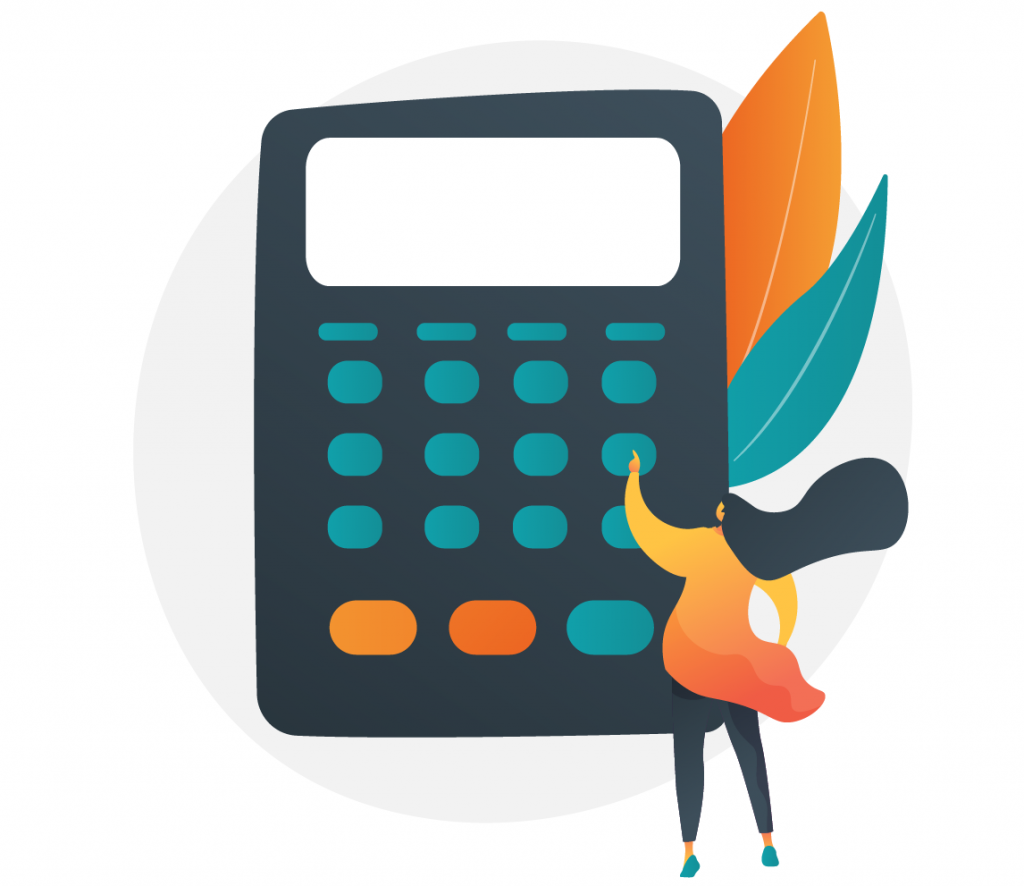# Sample Size Calculator

## Use Worthix's Sample Size Calculator for CX surveying in a few simple steps

### To calculate your sample size, simply open the Worthix Sample Size Calculator and insert the following numbers and percentages:Document

## How to determine the sample size?

To determine the sample size, you need 4 important factors: Population size, confidence level, margin of error and standard deviation.

Population Size — The total size of your population. This can be an approximation; it does not have to be exact. For example, if your survey needs to target video-game users between ages 13 to 25 in North and South America, your population size would be the approximate total number of video-game users across these continents.

Confidence Level — Confidence Levels works hand-in-hand with Margin of Error. It’s where you determine how confident (or mathematically correct) you want to be regarding your margin. The most common confidence levels are between 95% and 99% confident.

Margin of Error (Confidence Interval) — Mathematically, no sample is perfect. By determining a margin of error, you are deciding which percentage of error you’ll accept in your survey. The standard margin of error is between 3% and 5%.

Standard Deviation — This is used to measure how far your results may vary from the mean once you have collected and analyzed your data. For this calculator, we have pre-established the value at .5, since the calculation is pre-survey.